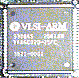# ShiftingThe ARM processor incorporates a barrel shifter that can be used with the data processing instructions (ADC, ADD, AND, BIC, CMN, CMP, EOR, MOV, MVN, ORR, RSB, SBC, SUB, TEQ, TST). You can also use the barrel shifter to affect the index value in LDR/STR operations.

There are six mnemonics for the different shift types:

```  LSL  Logical Shift Left
ASL  Arithmetic Shift Left
LSR  Logical Shift Right
ASR  Arithmetic Shift Right
ROR  Rotate Right
RRX  Rotate Right with Extend
```
`ASL` and `LSL` are the same, and may be freely interchanged.

You can specify a shift with an immediate value (from zero to thirty one), or by a register which contains a value between zero and thirty one.

## Logical or Arithmetic Shift Left

```  Rx, LSL #n    or
Rx, ASL #n    or
Rx, LSL Rn    or
Rx, ASL Rn```
The contents of Rx will be taken and shifted to a more significant position by the amount specified by 'n' or in the register Rn. The least significant bits are filled with zeroes. The high bits shifted off to the left are discarded, except for the notional bit thirty three (i.e., the last bit to be shifted off) which becomes the value of the carry flag on exit from the barrel shifter.

Consider the following:

```  MOV    R1, #12
MOV    R0, R1, LSL#2```
On exit, R0 is 48. The instructions forming the sum `R0 = #12, LSL#2` is equivalent to the BASIC `R0 = 12 << 2`

## Logical Shift Right

```  Rx, LSR #n    or
Rx, LSR Rn```
This does the notional opposite of shifting left. Everything is shifted to the right, to a less significant position. It is the same as `register = value >>> shift`.

## Arithmetic Shift Right

```  Rx, ASR #n    or
Rx, ASR Rn```
This is similar to LSR, with the exception that the high bits are filled with the value of bit 31 of the register being shifted (Rx), in order to preserve the sign in 2's complement maths. It is the same as `register = value >> shift`.

## Rotate Right

```  Rx, ROR #n    or
Rx, ROR Rn```
Rotate Right is similar to a Logical Shift Right, except the bits which would normally be shifted off the right are replaced on the left, this the bits 'rotate'. An operation where the amount to be shifted by is 32 would result in the output being identical to the input as all bits would have been shifted by 32 places, i.e., right back to where they started!

## Rotate Right with Extend

`  Rx, RRX`
This is a ROR#0 operation, which rotates one place to the right - the difference is that the processor's Carry flag is used to provide a 33 bit quantity to be shifted.
Thanks to Ian Jeffray for the correction.